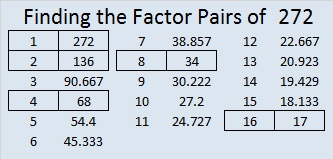# 272 and Level 4

• 272 is a composite number.
• Prime factorization: 272 = 2 x 2 x 2 x 2 x 17, which can be written (2^4) x 17
• The exponents in the prime factorization are 4 and 1. Adding one to each and multiplying we get (4 + 1)(1 + 1) = 5 x 2 = 10. Therefore 272 has 10 factors.
• Factors of 272: 1, 2, 4, 8, 16, 17, 34, 68, 136, 272
• Factor pairs: 272 = 1 x 272, 2 x 136, 4 x 68, 8 x 34, or 16 x 17
• Taking the factor pair with the largest square number factor, we get √272 = (√16)(√17) = 4√17 ≈ 16.492Give this Level 4 puzzle a try!Print the puzzles or type the factors on this excel file: 12 Factors 2014-10-20This site uses Akismet to reduce spam. Learn how your comment data is processed.